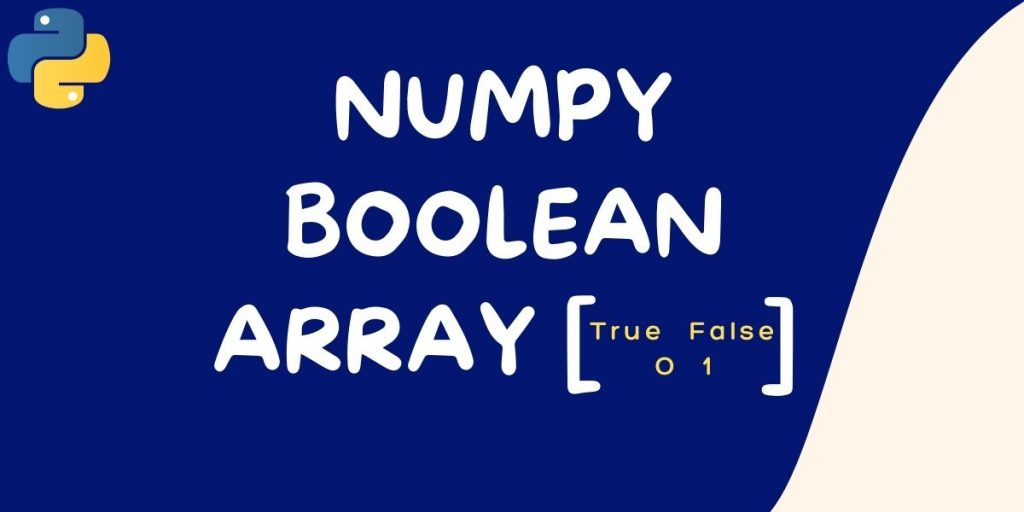# Numpy Boolean Array – Easy Guide for BeginnersThe Numpy boolean array is a type of array (collection of values) that can be used to represent logical ‘True’ or ‘False’ values stored in an array data structure in the Python programming language.

The use of a boolean array in conjunction with logic operators can be an effective way to reduce runtime computational requirements when a single logical value is needed from one or more complex variables. Boolean Arrays also find their usefulness in resultant arrays, on performing some operations.

While there may at first seem to be little use for such a construct, it is particularly important to beginners, who will often find themselves using boolean variables and arrays before they are familiar with other complex Python data types with greater flexibility.

Boolean Arrays in Python are implemented using the NumPy python library. Numpy contains a special data type called the
numpy.BooleanArray(count, dtype=bool) . This results in an array of bools(as opposed to bit integers) where the values are either 0 or 1.

Also read: Python – An Introduction to NumPy Arrays

## Declaring a Numpy Boolean Array

A boolean array can be made using dtype=bool, manually. All values other than ‘0’, ‘False’, ‘None’, or empty strings are considered True in a boolean array.

```import numpy as np
arr = np.array([5, 0.001, 1, 0, 'g', None, True, False, '' "], dtype=bool)
print(bool_arr)

#Output: [True True True False True False True False False]
```

### Numpy Boolean Array – Relational Operations

When relation operations are performed on the numpy boolean array, all the values are printed True where the condition matches, else other values are printed as False. Demonstrated in the below code example for equivalent operation, where values of the boolean array are checked for equal to 2.

```import numpy as np
A = np.array([2, 5, 7, 3, 2, 10, 2])
print(A == 2)

#Output: [True False False False True False True]
```

Relational Operations such as: “<“, “>”, “<=”, and “>=” works as well, for computation.

The operation works for higher dimension arrays as well:

```import numpy as np
# a 4x3 numpy array
A = np.array([[35, 67, 23, 90],   [89, 101, 55, 12],   [45, 2, 72, 33]])
print (A>=35)

#Output: [[ True  True  False  True] [ True  True  True False] [ True  False True False]]
```

Similarly, True/False can be replaced by 0/1, using the astype() object to convert it to int type.

```import numpy as np
A = np.array([[90, 11, 9, 2, 34, 3, 19, 100,  41], [21, 64, 12, 65, 14, 16, 10, 122, 11], [10, 5, 12, 15, 14, 16, 10, 12, 12], [ 49, 51, 60, 75, 43, 86, 25, 22, 30]])
B = A < 20
B.astype(np.int)

#Output: array([[0, 1, 1, 1, 0, 1, 1, 0, 0],       [0, 0, 1, 0, 1, 1, 1, 0, 1],       [1, 1, 1, 1, 1, 1, 1, 1, 1],       [0, 0, 0, 0, 0, 0, 0, 0, 0]])
```

Where 0 represents False and 1 represents True in the int type.

### Numpy Boolean Array – Logical Operations

Logical Operations such as: AND, OR, NOT, XOR is also operational on the boolean array with the following syntax method.

```numpy.logical_and(a,b)
numpy.logical_or(a,b)
numpy.logical_not(a,b)

# a and b are single variables or a list/array.

#Output: Boolean value
```

### Numpy Boolean Array Index

It is a property of Numpy that you can use to access specific values of an array using a boolean array. Also ready more about array indexing here.

```import numpy as np
# 1D Boolean indexing
A = np.array([1, 2, 3])B = np.array([True, False, True])
print(A[B])
# Output: [1, 3]

# 2D Boolean indexing
A = np.array([4, 3, 7],  [1, 2, 5])
B = np.array([True, False, True], [False, False, True])
print(A[B])

#Output: [4, 7, 5]
```

### Conclusion

Using Numpy’s Boolean array is a simple way to make sure that the contents of your array are what you expect them to be without having to inspect each element. Hope you have learned well about numpy boolean array, how to implement it and perform operations on it.